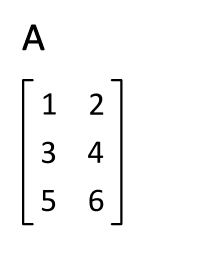# C Code To Find Transpose Of A Matrix

Hello Guys,
Today I'm gonna show you the C Code, to find transpose of a M x N Matrix.
The transpose of a matrix was introduced in 1858 by the British mathematician Arthur Cayley. We know that in linear algebra, the transpose of a matrix A is another matrix AT (a created by any one of the following actions:

• Reflect A over its main diagonal (which runs from top-left to bottom-right) to obtain AT
• Write the rows of A as the columns of AT
• Write the columns of A as the rows of AT

Formally, the i th row, j th column element of AT is the j th row, i th column element of A:
AT(i,j) = A(j,i)
If A is an m × n matrix then AT is an n × m matrix.

The same is reflected through this image:Transpose Of A Matrix

In simple terms,
if we have a matrix:
1 2
3 7
4 6

Its Transpose will be:
1 3 4
2 7 6

## Here is the C Code to find transpose of a matrix:

```/* Double-Click To Select Code */

#include<stdio.h>
#include<conio.h>

void main()
{
int m, n, c, d, matrix, transpose;
clrscr();
printf("Enter the number of rows and columns of the matrix a: \n");
scanf("%d%d",&m,&n);
printf("\nEnter the elements of matrix: \n");

for( c = 0 ; c < m ; c++ )
{
for( d = 0 ; d < n ; d++ )
{
printf("a[%d][%d] = ",m,n);
scanf("%d",&matrix[c][d]);
}
}

printf("\nOriginal Matrix: \n");
for( c = 0 ; c < m ; c++ )
{
for( d = 0 ; d < n ; d++ )
{
printf("%4d",matrix[c][d]);
}
printf("\n");
}

for( c = 0 ; c < m ; c++ )
{
for( d = 0 ; d < n ; d++ )
{
transpose[d][c] = matrix[c][d];
}
}

printf("\nTranspose of entered matrix: \n");

for( c = 0 ; c < n ; c++ )
{
for( d = 0 ; d < m ; d++ )
{
printf("%4d",transpose[c][d]);
}
printf("\n");
}
getch();
}```

## Output:

Please Comment If You Liked This Post !!